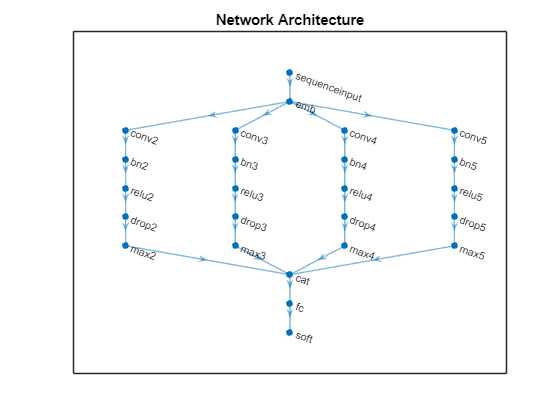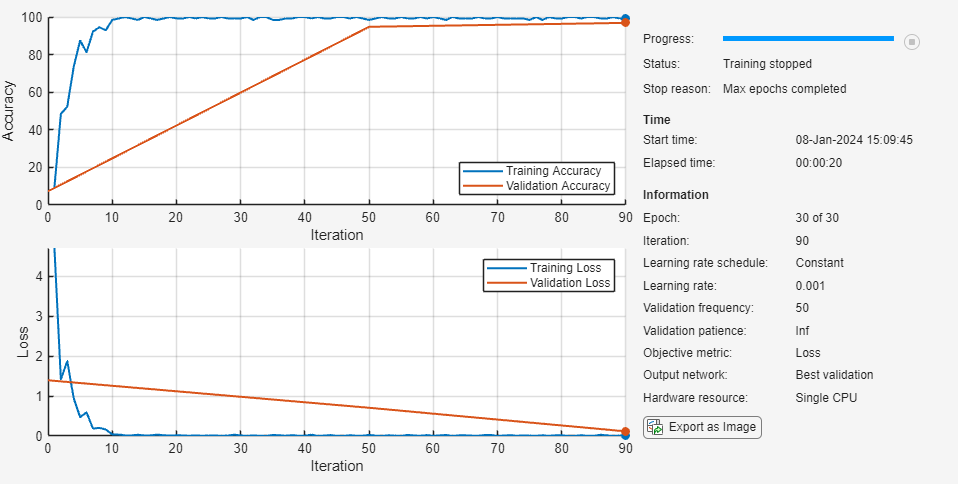# Classify Text Data Using Convolutional Neural Network

This example shows how to classify text data using a convolutional neural network.

To classify text data using convolutions, use 1-D convolutional layers that convolve over the time dimension of the input.

This example trains a network with 1-D convolutional filters of varying widths. The width of each filter corresponds the number of words the filter can see (the n-gram length). The network has multiple branches of convolutional layers, so it can use different n-gram lengths.

Create a tabular text datastore from the data in `factoryReports.csv` and view the first few reports.

```data = readtable("factoryReports.csv"); head(data)```
```ans=8×5 table Description Category Urgency Resolution Cost _______________________________________________________________________ ______________________ __________ ______________________ _____ {'Items are occasionally getting stuck in the scanner spools.' } {'Mechanical Failure'} {'Medium'} {'Readjust Machine' } 45 {'Loud rattling and banging sounds are coming from assembler pistons.'} {'Mechanical Failure'} {'Medium'} {'Readjust Machine' } 35 {'There are cuts to the power when starting the plant.' } {'Electronic Failure'} {'High' } {'Full Replacement' } 16200 {'Fried capacitors in the assembler.' } {'Electronic Failure'} {'High' } {'Replace Components'} 352 {'Mixer tripped the fuses.' } {'Electronic Failure'} {'Low' } {'Add to Watch List' } 55 {'Burst pipe in the constructing agent is spraying coolant.' } {'Leak' } {'High' } {'Replace Components'} 371 {'A fuse is blown in the mixer.' } {'Electronic Failure'} {'Low' } {'Replace Components'} 441 {'Things continue to tumble off of the belt.' } {'Mechanical Failure'} {'Low' } {'Readjust Machine' } 38 ```

Partition the data into training and validation partitions. Use 80% of the data for training and the remaining data for validation.

```cvp = cvpartition(data.Category,Holdout=0.2); dataTrain = data(training(cvp),:); dataValidation = data(test(cvp),:);```

### Preprocess Text Data

Extract the text data from the `"Description"` column of the table and preprocess it using the `preprocessText` function, listed in the section Preprocess Text Function of the example.

`documentsTrain = preprocessText(dataTrain.Description);`

Extract the labels from the `"Category"` column and convert them to categorical.

`TTrain = categorical(dataTrain.Category);`

View the class names and the number of observations.

`classNames = unique(TTrain)`
```classNames = 4×1 categorical Electronic Failure Leak Mechanical Failure Software Failure ```
`numObservations = numel(TTrain)`
```numObservations = 384 ```

Extract and preprocess the validation data using the same steps.

```documentsValidation = preprocessText(dataValidation.Description); TValidation = categorical(dataValidation.Category);```

### Convert Documents to Sequences

To input the documents into a neural network, use a word encoding to convert the documents into sequences of numeric indices.

Create a word encoding from the documents.

`enc = wordEncoding(documentsTrain);`

View the vocabulary size of the word encoding. The vocabulary size is the number of unique words of the word encoding.

`numWords = enc.NumWords`
```numWords = 436 ```

Convert the documents to sequences of integers using the `doc2sequence` function.

`XTrain = doc2sequence(enc,documentsTrain);`

Convert the validation documents to sequences using the word encoding created from the training data.

`XValidation = doc2sequence(enc,documentsValidation);`

### Define Network Architecture

Define the network architecture for the classification task.

The following steps describe the network architecture.

• Specify an input size of 1, which corresponds to the channel dimension of the integer sequence input.

• Embed the input using a word embedding of dimension 100.

• For the n-gram lengths 2, 3, 4, and 5, create blocks of layers containing a convolutional layer, a batch normalization layer, a ReLU layer, a dropout layer, and a max pooling layer.

• For each block, specify 200 convolutional filters of size 1-by-N and a global max pooling layer.

• Connect the input layer to each block and concatenate the outputs of the blocks using a concatenation layer.

• To classify the outputs, include a fully connected layer with output size K, a softmax layer, and a classification layer, where K is the number of classes.

Specify the network hyperparameters.

```embeddingDimension = 100; ngramLengths = [2 3 4 5]; numFilters = 200;```

First, create a layer graph containing the input layer and a word embedding layer of dimension 100. To help connect the word embedding layer to the convolution layers, set the word embedding layer name to `"emb"`. To check that the convolution layers do not convolve the sequences to have a length of zero during training, set the `MinLength` option to the length of the shortest sequence in the training data.

```minLength = min(doclength(documentsTrain)); layers = [ sequenceInputLayer(1,MinLength=minLength) wordEmbeddingLayer(embeddingDimension,numWords,Name="emb")]; lgraph = layerGraph(layers);```

For each of the n-gram lengths, create a block of 1-D convolution, batch normalization, ReLU, dropout, and 1-D global max pooling layers. Connect each block to the word embedding layer.

```numBlocks = numel(ngramLengths); for j = 1:numBlocks N = ngramLengths(j); block = [ convolution1dLayer(N,numFilters,Name="conv"+N,Padding="same") batchNormalizationLayer(Name="bn"+N) reluLayer(Name="relu"+N) dropoutLayer(0.2,Name="drop"+N) globalMaxPooling1dLayer(Name="max"+N)]; lgraph = addLayers(lgraph,block); lgraph = connectLayers(lgraph,"emb","conv"+N); end```

Add the concatenation layer, the fully connected layer, the softmax layer, and the classification layer.

```numClasses = numel(classNames); layers = [ concatenationLayer(1,numBlocks,Name="cat") fullyConnectedLayer(numClasses,Name="fc") softmaxLayer(Name="soft") classificationLayer(Name="classification")]; lgraph = addLayers(lgraph,layers);```

Connect the global max pooling layers to the concatenation layer and view the network architecture in a plot.

```for j = 1:numBlocks N = ngramLengths(j); lgraph = connectLayers(lgraph,"max"+N,"cat/in"+j); end figure plot(lgraph) title("Network Architecture")```### Train Network

Specify the training options:

• Train with a mini-batch size of 128.

• Validate the network using the validation data.

• Return the network with the lowest validation loss.

• Display the training progress plot and suppress the verbose output.

```options = trainingOptions("adam", ... MiniBatchSize=128, ... ValidationData={XValidation,TValidation}, ... OutputNetwork="best-validation-loss", ... Plots="training-progress", ... Verbose=false);```

Train the network using the `trainNetwork` function.

`net = trainNetwork(XTrain,TTrain,lgraph,options);`### Test Network

Classify the validation data using the trained network.

`YValidation = classify(net,XValidation);`

Visualize the predictions in a confusion chart.

```figure confusionchart(TValidation,YValidation)```Calculate the classification accuracy. The accuracy is the proportion of labels predicted correctly.

`accuracy = mean(TValidation == YValidation)`
```accuracy = 0.9375 ```

### Predict Using New Data

Classify the event type of three new reports. Create a string array containing the new reports.

```reportsNew = [ "Coolant is pooling underneath sorter." "Sorter blows fuses at start up." "There are some very loud rattling sounds coming from the assembler."];```

Preprocess the text data using the preprocessing steps as the training and validation documents.

```documentsNew = preprocessText(reportsNew); XNew = doc2sequence(enc,documentsNew);```

Classify the new sequences using the trained network.

`YNew = classify(net,XNew)`
```YNew = 3×1 categorical Leak Electronic Failure Mechanical Failure ```

### Preprocess Text Function

The `preprocessTextData` function takes text data as input and performs these steps:

1. Tokenize the text.

2. Convert the text to lowercase.

```function documents = preprocessText(textData) documents = tokenizedDocument(textData); documents = lower(documents); end```